# Introduction to Data Science with Python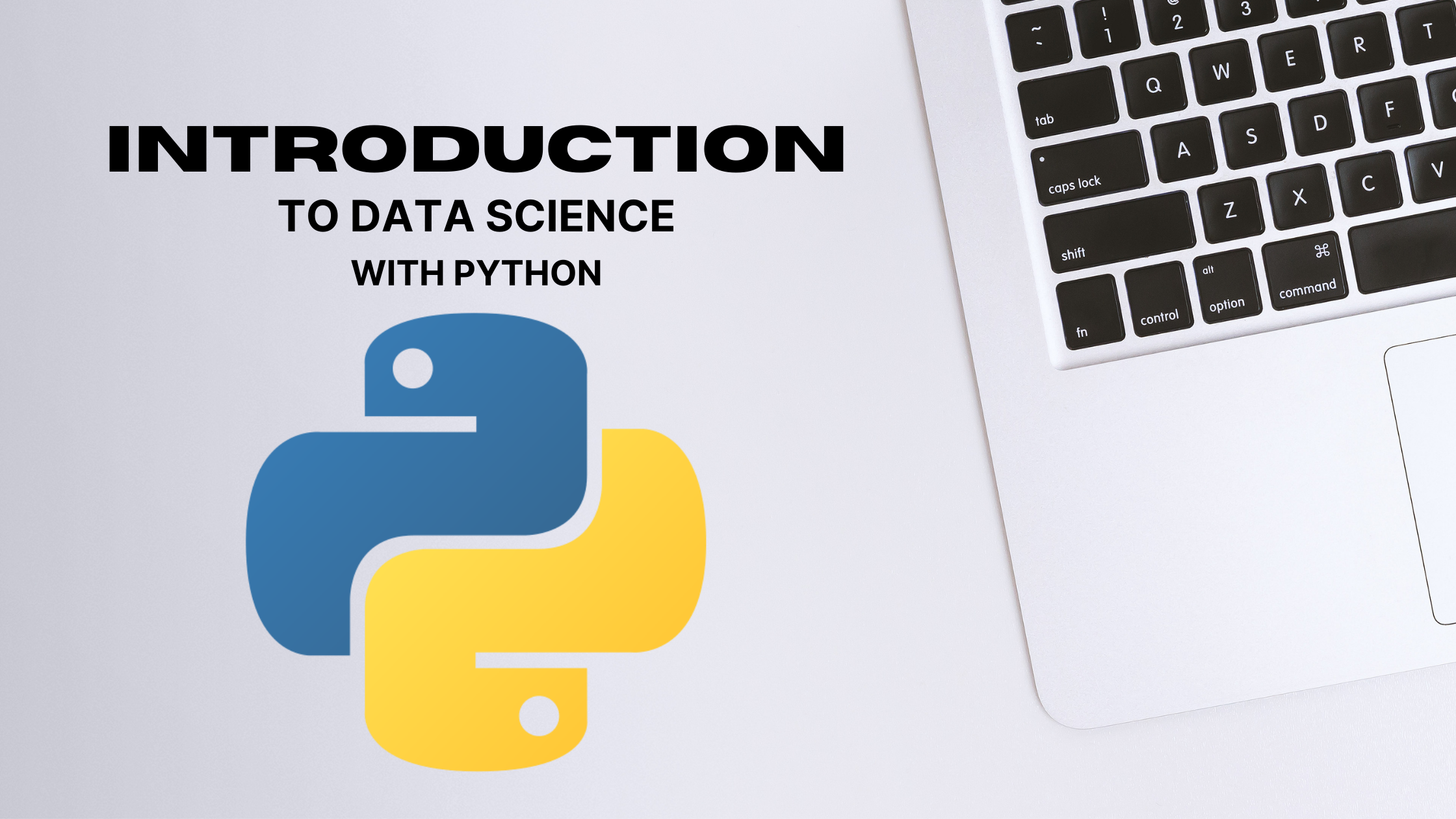Introduction to Data Science with Python

Python is a programming language intended for general-purpose programming and belongs to the category of high-level programming language.

As a general-purpose programming language, Python is used for a variety of problems such as web or mobile application development, data science, etc.

Python is included in the high-level programming language category because the Python programming language is easy to read and write by humans.

The Python programming language was created by Guido van Rossum and was first introduced in 1991 as an open-source project. The license from Python is open-source from Python, or in other words anyone can develop computer programs using the Python programming language for both commercial / non-commercial purposes.

List Library Python

1. Numpy (numerical python) is a library that makes it easy to define arrays either 1D, 2D, 3D or nD, and also has functions for linear algebra.

2. Scipy (Scientific Python) is a library that is intended for scientific computing purposes such as linear algebra, numerical integration and differentiation, Fourier transforms, optimization, interpolation, statistics and others.

3. Pandas is a library for data processing in tabular form (such as excel) which is a de facto library for data scientists in processing data from various sources such as CSV, TSV, Excel, SQL queries, Google BigQuery, SAS, Stata, SPSS, etc.

4. Matplotlib used for visualization of data into various 2D or 3D graph forms, such as line charts, bar charts, histograms, polar charts, error bar plots, and other types of charts.

5. Scikit-learn is a Scipy Toolkit aimed at generating predictive models using machine learning.

6. Seaborn is a library created from Matplotlib which is aimed at visualizing statistical graphics with attractive colors, well integrated with Pandas.

Python Language Structure

1. Statements : Instructions are given line by line to be executed by the program.

2. Variables : Identification used to store data or information.

3. Literals : Data or information used to fill a variable.

4. Operators : Symbols used to change the value of a variable by involving one or more variables and literals.

5. Reseved Words : A collection of words that have a special meaning in the Python programming language and cannot be used for variables and literals.

6. Whitespace : In Python, spaces and tabs have a special meaning to indicate a series of blocks in Python code.

7. Comments : A set of text written in a program that will not affect the results of a program.

Python “Hello World” & StatementInput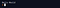Output

Note:

• “Hello World” called a statement.
• print () is the basic python function to print the given text

Variables in Python

Here are 3 important rules that must be followed when we declare a variable in Python.

1. The name of a variable must begin with the letter (a-z, A-Z) or an underscore underscore character (_) and cannot begin with a number (0–9).

2. Variables can only contain alphabetic characters and numbers and an underscore (a-z, A-Z, 0–9, _)

3. The variable is case-sensitive which means that the variables HIGH, height, and Height refer to three different variables.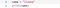Input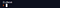Output

Comments in Python

Comments are a collection of text written in a program and will not affect the results of a program.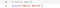Input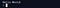Output

Data Enthusiast